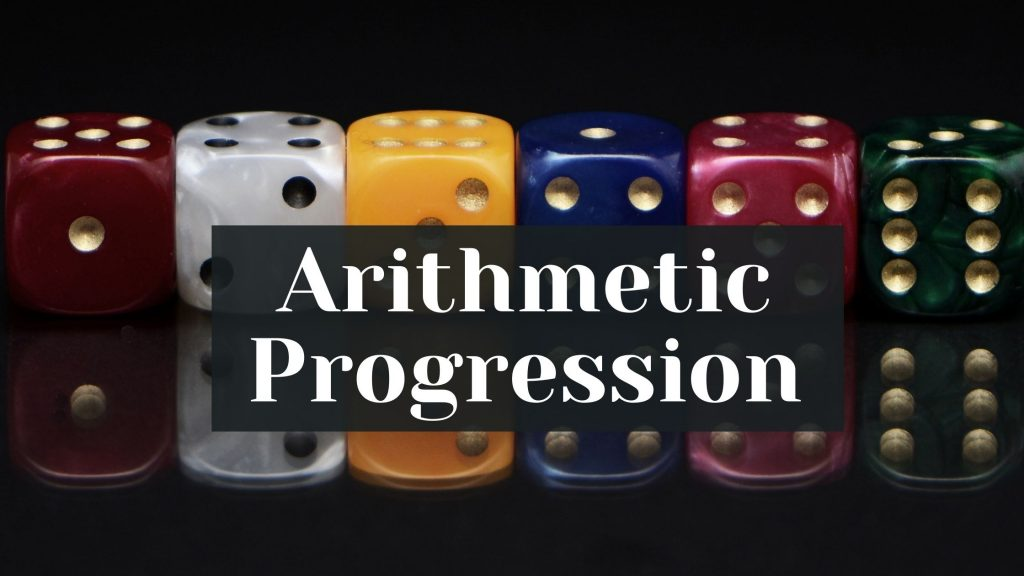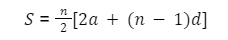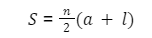Maths
Study Material

# Arithmetic Progression | Definition | Formulas | Numerical |

Arithmetic Progression (A.P) is a sequence of numbers where the difference between any two successive numbers is constant. Read here more about A.P and its formulas.

4 minutes longHesap Oluştur

Got stuck on homework? Get your step-by-step solutions from real tutors in minutes! 24/7. Unlimited.

An Arithmetic Progression (A.P)  is defined as a sequence of numbers in which for every pair of consecutive terms, we obtain the second number by adding a fixed number to the first one. Furthermore in this article, we’ve provided arithmetic progression definition along side all the AP formula with solved examples.

## What is Arithmetic Progression?

Arithmetic Progression (A.P) is a sequence of numbers where the difference between any two successive numbers is constant.

For example : 2, 4, 6, 8, 10……. is in a series which has a common difference (4 – 2) between two successive terms is equal to 2. If we take natural numbers as an example of series 1, 2, 3, 4…… then the common difference (2 – 1) between the two successive terms is equal to 1.

Therefore, arithmetic progression should be defined as “A mathematical sequence in which the difference between two consecutive terms is always a constant“.

#### In an Arithmetic Progression we need to focus on three main terms which will help us to solve problems:

• Common difference (d)
• nth Term (an)
• Sum of the first n terms (Sn)

## Common Difference

The common difference is the fixed constant whose value remains the same throughout the sequence. It is the difference between any two consecutive terms of the AP. So, the formula for common difference of an AP is:

Common Difference(d) = an+1 – an

## Formula for nth term of an Arithmetic Progression is:

an = a + (n-1)d

• a = First term
• d = Common difference
• n = Number of terms
• an = nth term

Let’s further understand this formula with an example:

For Example: Find the nth term of AP: 6, 9, 12, 15, 18, …, an, if the number of terms is 13.

Solution: AP: 6, 9, 12, 15, 18, …, an

(Given) n = 13

By the formula we know, an = a + (n – 1)d

First-term, a = 6

Common difference, d = (9 – 6) = 3

Therefore, an = 6 + (13 – 1)3 = 6 + 36 = 42

## Sum of n Terms of AP Formula

The sum of the first n terms can be calculated if the first term and the total number of terms are known .So, the formula for the sum of Arithmetic Progression is:• S = Sum of n terms of AP
• n = Total number of terms
• a = First term
• d = Common difference

Furthermore, let’s understand this formula with examples:

Example : Find the sum of the following arithmetic progression: 10, 15, 20, 25, … The total number of terms is 13.

Solution: AP = 10, 15, 20, 25, …

We have: a = 10, d = (15 – 10) = 5, and n = 13

By the AP sum formula, therefore:

S = n/2[2a + (n − 1) × d]

= 14/2[2 x 10 + (13 – 1) x 5]= 14/2[20 + 60] = 14/2  = 7 x 80 = 560

Hence, the sum of the AP is 560.

## Arithmetic Progression Sum Formula When First and Last Terms are Given:

When the first and last term of an AP is given, we can calculate the sum of the AP by using this formula:Example : Find the sum of the following AP: 15, 19, 23, 27, … , 75.

Solution: AP: 15, 19, 23, 27, … , 75

We have: a = 15, d = (19 – 15) = 4, and l = 75

We have to find n. So, using the formula: l = a + (n – 1)d, we get

75 = 15 + (n – 1) x 4

60 = (n – 1) x 4

n – 1 = 15

n = 16

Here the first and last terms are given, so by the AP sum formula, we know:

S = n/2[first term + last term]

Hence, Substituting the values, we get:

S = 16/2 [15 + 75]= 8 x 90

= 720

Hence, the sum of the AP is 720.

## Picked For You

Furthermore, these are some topics which might interest you: